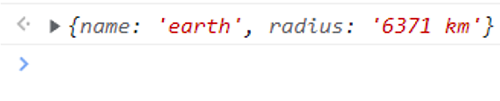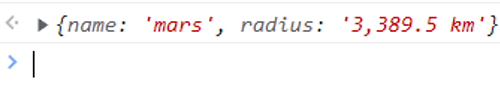# How can I merge properties of two JavaScript objects dynamically?

Learn how can I merge properties of two JavaScript objects dynamically?
Submitted by Pratishtha Saxena, on April 28, 2022

## What are JavaScript Objects?

JavaScript objects are variables containing a single value or many values.

For example:

```let planet = "Earth";
```

Here, the planet is an object. It can also be written as follows with many value pairs.

```let planet = {name: "Earth", radius: "6371 km", age: "4.543 billion years"}
```

It is represented by (), i.e., three dots. Spread operator in JavaScript allows you to quickly copy all or part of an existing array/object into another array/object.

You can use spread operator to combine or merge arrays/objects in JavaScript. It merges all the properties of all the objects in new object, but same properties will be replaced or overwritten by the last object.

For example: let there be two objects (planet_1 and planet_2) with some properties. They are then merged in the third object called planet.

Below is the JavaScript Code:

Example 1:

```let planet_1 = {
name: "earth",
}

let planet_2 = {
}

let planet = {
...planet_1,
...planet_2
}

planet
```

Output:Example 2:

```let planet_1 = {
name: "earth",
}

let planet_2 = {
name: "mars",
}

let planet = {
...planet_1,
...planet_2
}

planet
```

Output: